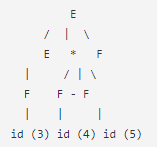Courses

# Predictive Top Down Parsing

## 10 Questions MCQ Test Compiler Design | Predictive Top Down Parsing

Description
This mock test of Predictive Top Down Parsing for Computer Science Engineering (CSE) helps you for every Computer Science Engineering (CSE) entrance exam. This contains 10 Multiple Choice Questions for Computer Science Engineering (CSE) Predictive Top Down Parsing (mcq) to study with solutions a complete question bank. The solved questions answers in this Predictive Top Down Parsing quiz give you a good mix of easy questions and tough questions. Computer Science Engineering (CSE) students definitely take this Predictive Top Down Parsing exercise for a better result in the exam. You can find other Predictive Top Down Parsing extra questions, long questions & short questions for Computer Science Engineering (CSE) on EduRev as well by searching above.
QUESTION: 1

### S → C C C → c C | d The grammar is

Solution:

Explanation: Since there is no conflict, the grammar is LL (1) hence a predictive parse table with no conflicts can be constructed.

QUESTION: 2

### Which of the following statements is false?

Solution:

Explanation:
If a grammar has more than one leftmost (or rightmost) derivation the grammar is ambiguous.

QUESTION: 3

### Which of the following derivations does a top-down parser use while parsing an input string?

Solution:

Explanation: Left to right constructing leftmost derivation of the sentence.

QUESTION: 4

Given the following expression grammar:E -> E * F | F + E | FF -> F – F | idWhich of the following is true?

Solution:QUESTION: 5

Which one of the following is true at any valid state in shift-reduce parsing?

Solution:

Explanation: The prefixes on the stack of a shift-reduce parser are called viable prefixes.

QUESTION: 6

In the context of abstract-syntax-tree and control-flow-graph.Which one of the following is true?

Solution:

Explanation: Successors depends on input .

QUESTION: 7

List-I                                List-II
A. Lexical analysis           1. Graph coloring
B. Parsing                         2. DFA minimization
C. Register allocation       3. Post-order traversal
D. Expression evaluation  4. Production tree

A B C D

Solution:

Explanation: The entire column an items matches the Column B items in a certain way.

QUESTION: 8

Which of the following pairs is the most powerful?

Solution:

Explanation parser algorithm is simple.

QUESTION: 9

Consider the following grammar G.
S → F ⎪ H
F → p ⎪ c
H → d ⎪ c
Which one is true?
S1: All strings generated by G can be parsed with help of LL (1).

S2: All strings generated by G can be parsed with help of LR (1).

Solution:

Explanation: There is ambiguity as the string can be derived in 2 possible ways.
First Leftmost Derivation
S → F
F → c
Second Leftmost Derivation
S → H
H → c

QUESTION: 10

What is the maximum number of reduce moves that can be taken by a bottom-up parser for a grammar with no epsilon- and unit-production to parse a string with n tokens?

Solution:

Explanation: the moves are n-1.